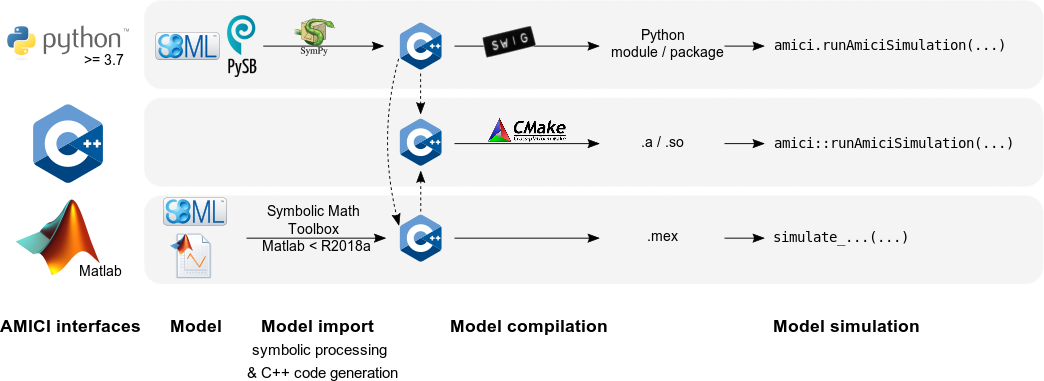AMICI provides a multi-language (Python, C++, Matlab) interface to the solvers (for s) and (for s). AMICI allows the user to read differential equation models specified as or and automatically compiles such models into Python modules, C++ libraries or .mex simulation files (Matlab).

In contrast to the (no longer maintained) sundialsTB Matlab interface, all necessary functions are transformed into native C++ code, which allows for a significantly faster simulation.

Beyond forward integration, the compiled simulation file also allows for forward sensitivity analysis, steady state sensitivity analysis and adjoint sensitivity analysis for likelihood-based output functions.

The interface was designed to provide routines for efficient gradient computation in parameter estimation of biochemical reaction models but it is also applicable to a wider range of differential equation constrained optimization problems.

## Features¶

• import

• import

• Generation of C++ code for model simulation and sensitivity computation

• Python, C++, Matlab interface

• Sensitivity analysis

• forward

• first- and second-order (second-order Matlab-only)

• Pre-equilibration and pre-simulation conditions

• Support for discrete events and logical operations

## Interfaces & workflow¶

The AMICI workflow starts with importing a model from either (Matlab, Python), (Python), or a Matlab definition of the model (Matlab-only). From this input, all equations for model simulation are derived symbolically and C++ code is generated. This code is then compiled into a C++ library, a Python module, or a Matlab .mex file and is then used for model simulation.The functionality of the Python, Matlab and C++ interfaces slightly differ, as shown in the following table:

Feature \ Interface

Python

C++

Matlab

import

yes ()

no

yes (<=R2017b)

import

yes

no

no

import

no

no

yes

Forward sensitivities

yes

yes

yes

yes

yes

yes

yes

yes

yes

Second-order sensitivities

no

no

yes

Events

yes

yes

yes

yes

yes

yes

yes

yes

no